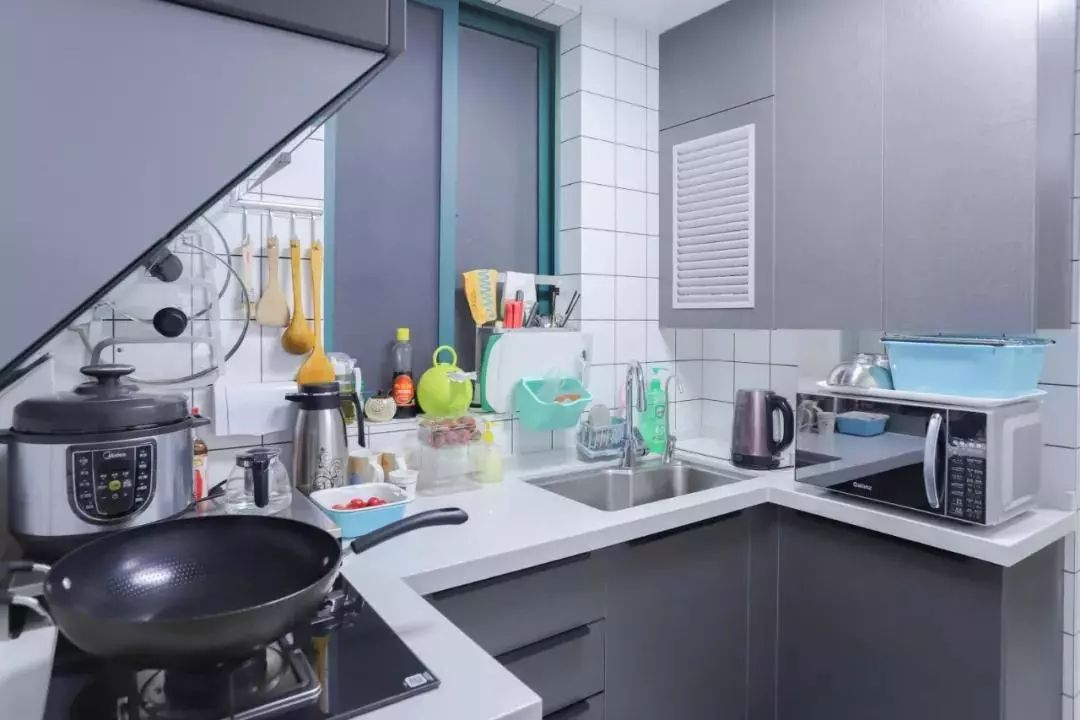﻿ 你家的厨房，千万不要这样装 - 承德门户网站

# 你家的厨房，千万不要这样装12' data-lazy='1' data-height='600' data-width='900' width='900' height='auto'>

3' data-lazy='1' data-height='770' data-width='814' width='814' height='auto'>

4' data-lazy='1' data-height='600' data-width='900' width='900' height='auto'>

5' data-lazy='1' data-height='310' data-width='408' width='408' height='auto'>' data-lazy='1' data-height='331' data-width='432' width='432' height='auto'>

6' data-lazy='1' data-height='480' data-width='640' width='640' height='auto'>

7

8' data-lazy='1' data-height='520' data-width='519' width='519' height='auto'>